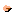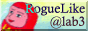# - MAngband Yorugao Original -## \$BJQ992U=j(B

• \$B6[D%46\$r;}B3\$9\$k\$?\$a0lDj;~4V\$G%f%K!<%/%b%s%9%?!<\$,I|3h\$9\$k!%(B(Thanks to D12014)
• \$B>/\$J\$\$%"!<%F%#%U%!%/%H\$rJd\$&\$?\$a2?\$B\$"\$k0lDj\$N3NN(\$G\$h\$j6/NO\$J%(%4%"%\$%F%`\$,=P8=\$9\$k!%(B(Thanks to D12014)
• recall \$B\$G9_\$j\$k3,\$rA*Br\$G\$-\$k!%(B(Thanks to D12014)
• \$B%b%s%9%?!<\$NKbK!5Z\$S%V%l%9\$N%@%a!<%8\$r\$r>/\$J\$/\$7\$F\$\$\$k!%(B \$B!J:#8=:_(B50\$B!s%+%C%H!K(B(Thanks to D12014)
• \$B>\$4T\$5\$l\$k%b%s%9%?!<\$N?t\$r8:\$i\$7\$F\$\$\$k!J:#8=:_(B50\$B!s%+%C%H!K(B
• \$B;CDj\$J\$,\$i%Q!<%F%#!<%^%8%C%/\$r%5%]!<%H(B(Thanks to D12014)
• Potion of Healing \$B5Z\$S(B Scroll of *Identify \$B\$rE9\$G9XF~\$G\$-\$k\$h\$&\$K\$7\$?!%(B

## \$BDI2C%"%\$%F%`(B

### \$B%(%4%"%\$%F%`(B

 Gauntlets of Weasteress +1d2 STR CON DEX, Free Action Prob: 1 in 33 Gauntles of Extra Attack +1d2 Extra Attack, Free Action Prob: 2 in 33 Weapon of the Edain +1d4 STR, Slay Dragon, Sustain STR, FreeAction Prob:5 in 175 Weapon of the Ents +1d4 CON, Slay Evil, Sustain CON, Regenerate Prob:5 in 175 Weapon of the Halfling +1d4 DEX, Slay Orc Troll, Sustain DEX, Slow Digestion Prob:5 in 175 Weapon of the Istari +1d5 INT, Slay Undead, One High Resist, Sustain INT, See Invisible Prob:5 in 175 Weapon of the Slaying +1d2 STR DEX, One Random Slay Prob:10 in 175 Weapon of the Miar +1 All Stats, One Random Immunity, Slay Evil Prob:1 in 141 Shooting Weapon of Ithilien +1d3 INT DEX, Extra Might Prob:1 in 21 Armor of the Phoenix Immunity to Fire, See Invisible, Feather Fall, Regenerate, Free Action Prob:4 in 129 Armor of the Valar +1 All Stats, One Random Sustain Prob:4 in 129 Armor of the Ainur +1d2 All Stats, One High Resist Prob:1 in 129 Shield of the Dragon One High Resist, Resist Fire, Cold, Acid, Lightning Prob:1 in 12 Boots of Woses +1d4 Stealth, One High Resist, Regenerate, Slow Digestio Prob:3 in 27 Rod of Stone to Mud Mage \$B7O0J30\$OI,?\!*!)(B Amulet of Regeneration \$B%j%"%k%?%\$%`\$J\$?\$aHs>o\$K=EMW(B Amulet of the Cleric One Power, Resist Fire, Cold, Acid, Lightning

### \$B%"!<%F%#%U%!%/%H(B

 The Pair of Soft Leather Boots of Tom Bombadil +3 Speed Stealth, Resist Blindness, See Invisible The Long Bow of Faramir +2 INT DEX, Resist Blindness, Regenerate, Slow Digestion, Extra Shot

## D12014\$B\$5\$s\$N%Q%C%A\$K\$h\$k%"%\$%F%`6/2=(B

• \$B1JB3\$N%m!<%V!&%(%k%U\$N3;!&%"%^%s\$N%/%m!<%/!&%I%i%4%s\$N=b\$K\$O!"(B3\$B\$D\$^\$G>e0LBQ@-\$,\$D\$/\$3\$H\$,\$"\$k!#(B
• \$B8-(\$B@;@o\$BG=NOCM=\$@5\$5\$l\$k%"%\$%F%`\$N>e8B\$O!"5)\$KDL>o\$h\$j(B(1d2)\$BB?\$/\$J\$k!#(B
• to-hit,to-dam,to-AC\$B\$X\$N%\!<%J%9CM\$O!"DL>o\$h\$jBg\$-\$\$!#(B
• \$B5)\$K;@\$G=}\$D\$+\$J\$/\$J\$k%"%\$%F%`\$,@8\$l\$k\$3\$H\$,\$"\$k!#(B

## \$BLdBjE@(B

\$B7G<(HD\$J\$I\$GOCBj\$K\$J\$C\$?\$b\$N\$r\$\\$A\$\\$A\$^\$H\$a\$F\$\$\$-\$^\$9!%(B
• mage \$BM-Mx\$NAuHw\$,B?\$\$(B
Alexiel \$B\$5\$s\$J\$I\$O\$=\$l\$[\$ILdBj\$J\$\$\$H\$*\$C\$7\$c\$F\$\$\$k!%(B
• Extra Attack \$B>.
\$BEv=i\$O@o;N\$NAuHw\$NA*Br;h\$rA}\$d\$9\$D\$b\$j\$G!\$(BFree Action \$B\$OIU\$1\$J\$\$(B \$B\$D\$b\$j\$@\$C\$?\$N\$G\$9\$,!\$>.
• Ithilien \$B\$J\$I(B
\$B\$=\$b\$=\$b\$J\$s\$G5]\$GCNG=(B(Int)\$B\$,>e\$,\$k\$N\$+\$b5?Ld\$G\$"\$k!%(B Amulet(Ring) of Cleric(Holliness) \$B\$J\$I\$rF3F~\$7\$FIT8xJ?46\$r\$J\$/\$9!)(B
• Potion of Healing \$B\$NHNGd(B
\$BANN77O\$NFCE5\$N0l\$D\$,>C\$(\$F\$\$\$k\$?\$a
• \$B%^%k%A%W%l%\$\$N3Z\$7\$_(B
\$B\$=\$b\$=\$b!\$(BAngband \$B\$O6(NO7?%^%k%A%W%l%\$\$K\$O8~\$\$\$F\$J\$\$\$H;W\$&!%(B \$B\$7\$+\$7!\$\$J\$s\$i\$+\$N2~B\$\$K\$h\$j\$=\$l\$i\$N3Z\$7\$_\$bA}\$(\$k\$H;W\$o\$l\$k!%(B
• \$B7P83CM%7%9%F%`(B
\$B8=:_\$OF10l%Q!<%F%#!<\$N?M\$,F1\$83,\$K\$\$\$k\$H\$-!\$:G9b%l%Y%k\$N%-%c%i%/%?(B \$B\$,E]\$7\$?\$b\$N\$H\$_\$J\$7\$F7P83CM\$r7W;;(B(\$B!)(B)\$B\$7\$FF,3d\$j\$7\$F\$\$\$k!%\$H\$j\$"\$(\$:(B \$B\$3\$l\$r\$b\$&>/\$77P83CM\$,F~\$k\$h\$&\$K\$K\$9\$k\$@\$1\$G\$b!\$\$A\$g\$C\$H\$^\$7\$K\$J\$j(B \$B\$=\$&!%\$H;W\$C\$?\$,\$=\$&\$9\$k\$H!\$(B \$BDc%l%Y%k\$J?M\$O\$=\$N3,\$G\$\$\$k\$@\$1\$G7P83CM\$,\$I\$s\$I\$s\$O\$\$\$j%l%Y%k\$,(B \$B\$"\$,\$j\$^\$/\$k\$H\$\$\$&J@32\$,!D(B \$B\$=\$N?M\$N%l%Y%k\$J\$j\$K7P83CM\$,F~\$k\$h\$&\$J(B \$B%7%9%F%`\$K\$9\$kI,MW\$,\$"\$j\$=\$&!%\$"\$H!\$Dc%l%Y%k\$N;~\$KKbK!=q(B(\$B5'Ex=q(B) \$BFI\$s\$G%l%Y%k%"%C%W\$9\$k\$N\$b\$I\$&\$+\$H;W\$&!%(B
• \$B%"!<%F%#%U%!%/%H(B
• \$BJ#?t2=(B
\$B\$d\$O\$jDq93\$"\$k!%\$h\$j6/NO\$J%(%4%"%\$%F%`\$,=P\$k\$N\$G\$=\$l\$[\$I(B \$B94\$i\$J\$/\$F\$b\$h\$/\$J\$C\$F\$-\$?\$h\$&\$J5\$\$,\$9\$k!%(B
• \$BKbK!(B
• \$B%-%c%i%/%?F1;N3NG'(B
• \$B\$*6b\$N;H\$\$F;(B
\$BD.\$NE92~B\$0F\$,=P\$F\$\$\$k!%(B
• \$BKA81\$NC1D4@-(B
• \$BMM!9\$JFC
• \$B%5%V%-%c%i(B or \$B2H(B
\$B2H\$h\$j\$b%5%V%-%c%i\$K;}\$?\$;\$kJ}\$,0BA4\$G\$"\$j!\$2H\$NB8:_0U5A\$,\$J\$\$!%(B \$B%5%V%-%c%i\$N;HMQ\$H\$\$\$&\$N\$O\$3\$Nl\$7\$N\$.\$J(B \$B2r7hK!\$G\$"\$k!%\$=\$N\$?\$a\$K%"%\$%F%`\$,>C\$(\$k\$N\$r\$J\$/\$5\$M\$P!D(B(\$BD.\$N(B \$B%"%\$%F%`\$OBP>]30\$K\$9\$k\$H\$+\$7\$F(B)\$B!%(BAngband \$B\$N\$h\$&\$J2H\$K\$9\$l\$P\$H\$\$\$&0F\$b!D(B
• \$B%5!<%P%j%;%C%H(B
• \$B0l2s8B\$j(B
• \$B2?2s\$b(B(\$BH>G/\$/\$i\$\$(B?)
\$B8D?ME*\$K\$O\$3\$NJ}<0\$G\$\$\$-\$?\$\$\$H;W\$C\$F\$\$\$k(B
• \$BH?BP(B

## To Do

\$B\$"\$H\$d\$k\$Y\$-\$3\$H\$O(B......
• \$BB<@5\$J\$I\$NF|K\\$C\$]\$\$%"!<%F%#%U%!%/%H\$rDI2C(B(\$B@hAw\$j(B)
• \$B>\:Y\$J%m%0>pJs\$r\$H\$k(B
• \$B%"!<%F%#%U%!%/%H\$rC/\$,=&\$C\$?\$+\$rI=<((B(\$B%f%K!<%/%b%s%9%?!<\$N\$h\$&\$K(B)
\$BMWK>\$J\$I\$,\$"\$j\$^\$7\$?\$i7G<(HD\$K=q\$\$\$F\$b\$i\$(\$k\$H\$"\$j\$,\$?\$\$\$G\$9!%(B(^_^;

\$B!Z%H%C%W%Z!<%8\$KLa\$k![(B \$B!Z(BMAngband \$B\$N%Z!<%8\$KLa\$k![(B